### Home > PC > Chapter 4 > Lesson 4.1.2 > Problem4-25

4-25.
1. Given: cos θ =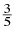. Homework Help ✎

1. Draw a right triangle and place values on the sides so that cos θ =.

2. Draw a unit circle diagram to clearly show the TWO possible positions for θ.

3. Use the Fundamental Pythagorean Identity or a right triangle to find all possible values for sin θ.

4. Find all possible values for tan θ.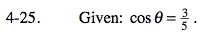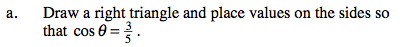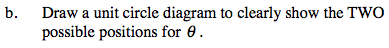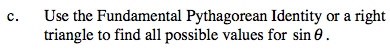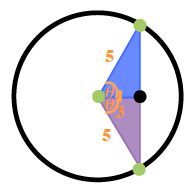It must be a 3-4-5 triangle because of the Pythagorean Theorem. Determine the two sine values.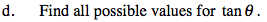$\tan(\theta)=\frac{\text{opposite}}\text{{adjacent}}=\frac{\pm4}{3}=\pm\frac{4}{3}$